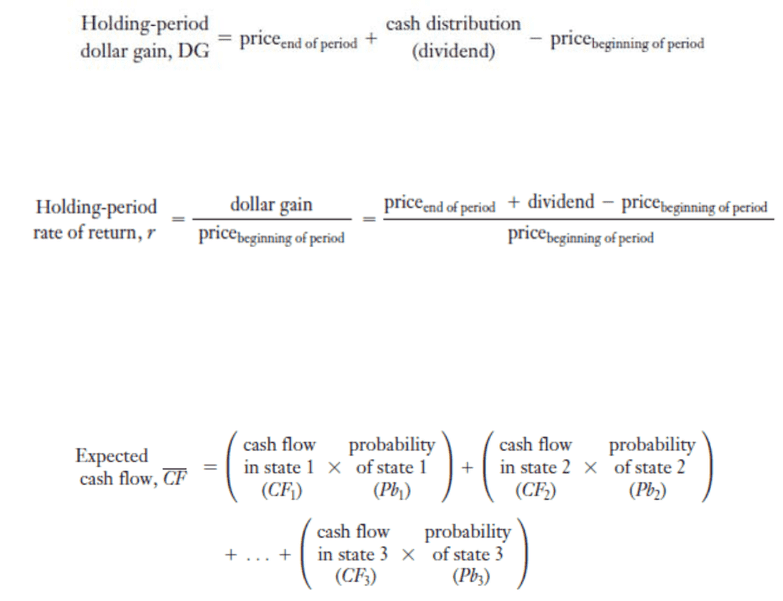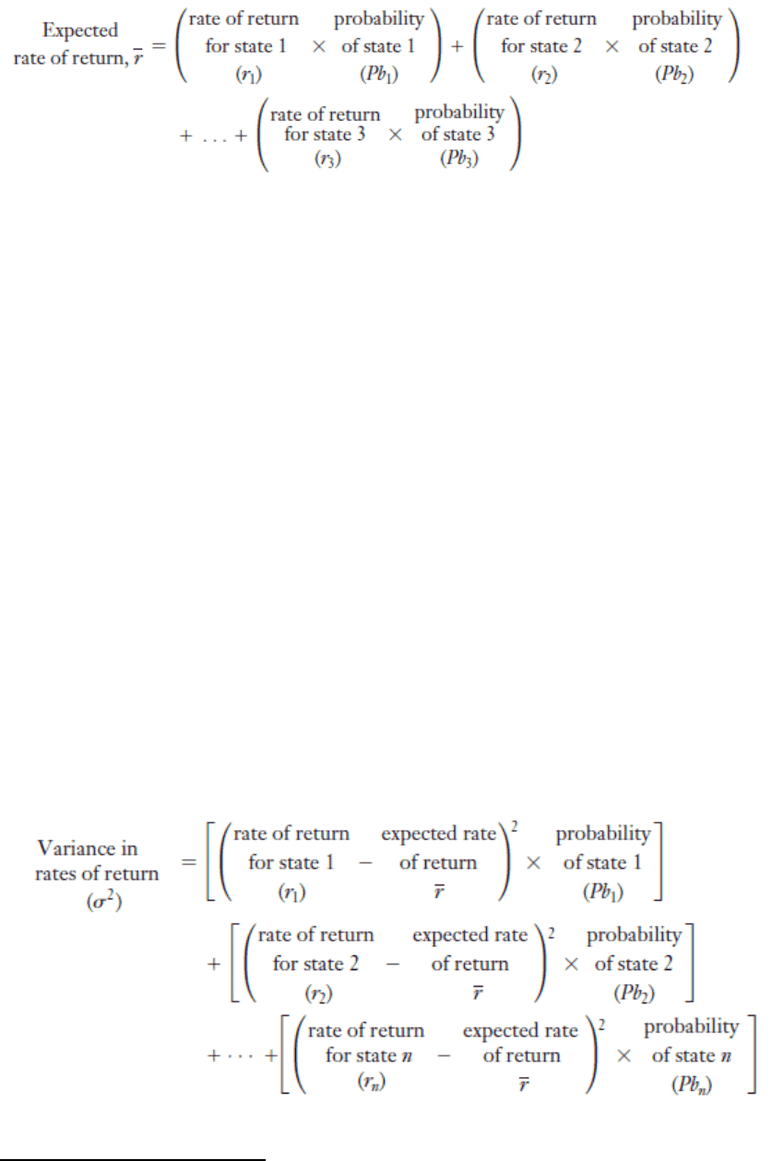Textbook Notes (280,000)
US (100,000)
LSU (20,000)
FIN (100)
A L L (10)
Chapter 6

FIN 3715 Chapter Notes - Chapter 6: Market Risk, Standard Deviation, Capital Asset

Department
Finance
Course Code
FIN 3715
Professor
A L L
Chapter
6

This preview shows pages 1-2. to view the full 6 pages of the document.Chapter 6
The Meaning and Measurement of Risk Return
To calculate the Holding-period dollar gain, you take the price at the end of the period plus the cash
distribution (dividend) and subtract the price at the beginning of the period. The formula is shown
below.
If you want to calculate the holding-period rate of return (also called the historical or realized rate of
return), you would use the formula below.
Investors face daily the risk return trade off of what they expect an investment to earn for them in the
future. They are not so concerned with the realized rate of return.
When looking at the expected cash flow, you need to remember that it is only the weighted average of
the cash flow that is possible. You can expect cash flows from various investments, however you need to
remember that there are numerous possible outcomes from the investments that you made. To
calculate the expected cash flow, you would use the formula below; adding as many states as needed.
It is important to remember that the expected rate of return is simply a way to identify the return on
investment, while factoring in risks. It also lets the investor know what the minimum return they should
accept. The three things you need to consider when calculating the rate of return is (1) the return in the
market overall, (2) the rate you would get if you invested in no risk options, and (3) the overall cost.
Many define rate of return as the weighted average of all conceivable outcomes, weighted by the
probability that it will actually happen. The formula is shown below.

Only pages 1-2 are available for preview. Some parts have been intentionally blurred.Solon, an Athenian statesman who was known to be one of the Seven Wise Men of Greece of that
period stated it perfectly:
There is risk in everything that one does, and no one knows where he will make his landfall
when his enterprise is at its beginning. One man, trying to act effectively, fails to foresee
something and falls into great and grim ruination, but to another man, one who is acting
ineffectively, a god gives good fortune in everything and escape from his folly.”1
When considering the amount of risk in an investment, you want to consider three important things:
1. What constitutes as risk?
2. How can we calculate the risk for each specific investment we make?
3. Will risk be reduced if we spread out our investments and diversify?
While we want to acknowledge that there are different kinds of risks, we don’t want to limit what we
invest in because of it. Risk is the unpredictability of cash flow in the future. The more possible
situations that can happen, the more risk increases.
One way we can quantify the risks involved in an investment is by figuring out the Standard Deviation, or
variance in rates of return. Standard Deviation has been simply defined as the measure of spread of the
numbers in a set of data from its mean value. It can also be said to be a measure of the variability or
volatility of any given date set. The formula to calculate the standard deviation is shown below.
There are five-steps to calculate the Standard Deviation as shown below:
1From Iambi et Elegi Graeci ante Alexandrum Cantati, vol. 2. Edited by M.L. West, translated by Arthur W.H. Adkins. (Oxford: Clarendon Press, 1972)
You're Reading a Preview

Unlock to view full version